Next: Moments of the Kinetic Up: Plasma Fluid Theory Previous: Moments of the Distribution

# Moments of the Collision Operator

Boltzmann's famous collision operator for a neutral gas considers only binary collisions, and is, therefore, bilinear in the distribution functions of the two colliding species: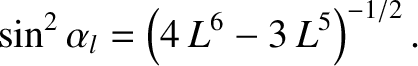(192)

where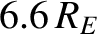is linear in each of its arguments. Unfortunately, such bilinearity is not strictly valid for the case of Coulomb collisions in a plasma. Because of the long-range nature of the Coulomb interaction, the closest analogue to ordinary two-particle interaction is mediated by Debye shielding, an intrinsically many-body effect. Fortunately, the departure from bilinearity is logarithmic in a weakly coupled plasma, and can, therefore, be neglected to a fairly good approximation (since a logarithm is a comparatively weakly varying function). Thus, from now on,is presumed to be bilinear.

It is important to realize that there is no simple relationship between the quantity, which describes the effect on species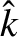of collisions with species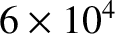, and the quantity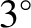. The two operators can have quite different mathematical forms (for example, where the masses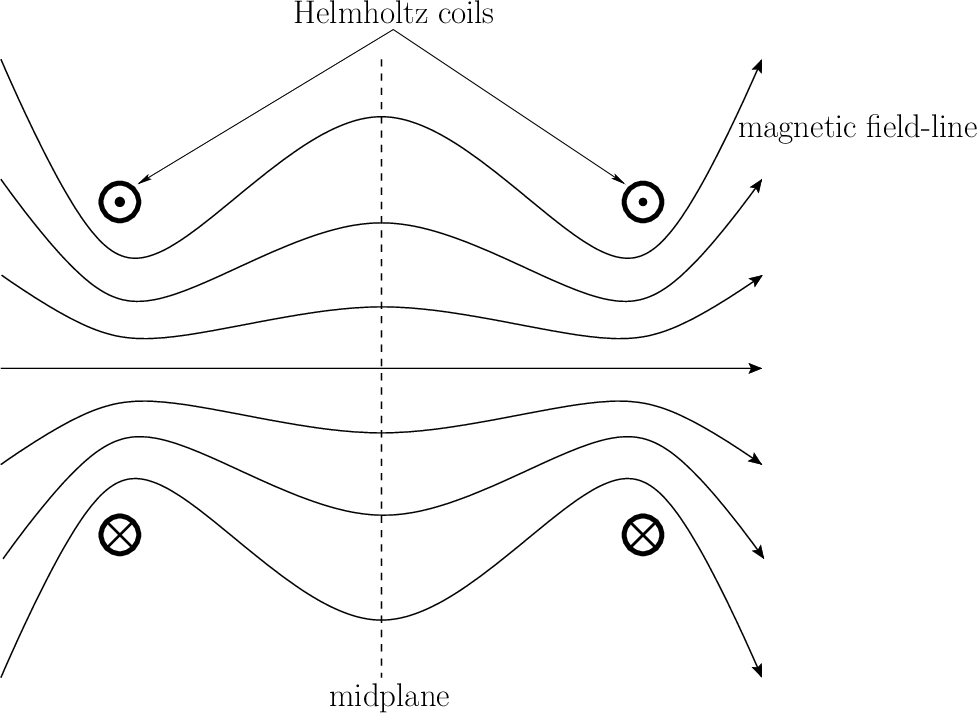and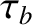are disparate), and they appear in different equations.

Neutral particle collisions are characterized by Boltzmann's collisional conservation laws: the collisional process conserves particles, momentum, and energy at each point. We expect the same local conservation laws to hold for Coulomb collisions in a plasma: the maximum range of the Coulomb force in a plasma is the Debye length, which is assumed to be vanishingly small.

Collisional particle conservation is expressed by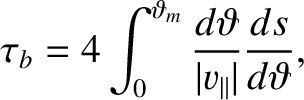(193)

Collisional momentum conservation requires that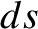(194)

That is, the net momentum exchanged between speciesandmust vanish. It is useful to introduce the rate of collisional momentum exchange, called the collisional friction force, or simply the friction force: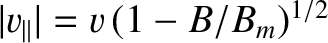(195)

Clearly,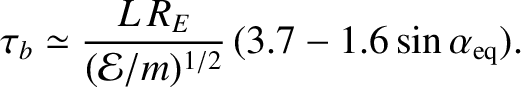is the momentum-moment of the collision operator. The total friction force experienced by speciesis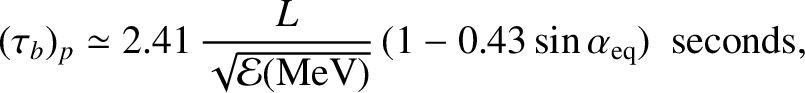(196)

Momentum conservation is expressed in detailed form as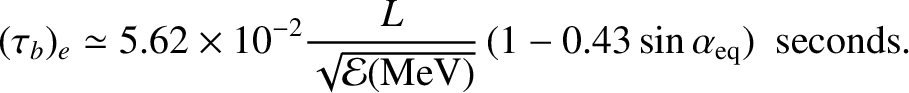(197)

and in non-detailed form as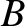(198)

Collisional energy conservation requires the quantity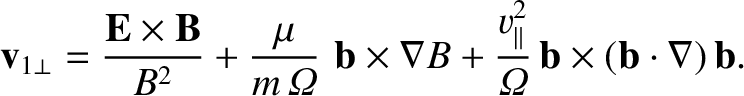(199)

to be conserved in collisions: i.e.,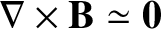(200)

Here, the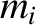-subscript indicates that the kinetic energy of both species is measured in the same lab'' frame. Because of Galilean invariance, the choice of this common reference frame does not matter.

An alternative collisional energy-moment is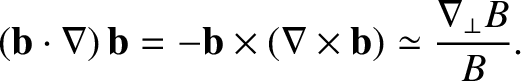(201)

i.e., the kinetic energy change experienced by species, due to collisions with species, measured in the rest frame of species. The total energy change for speciesis, of course,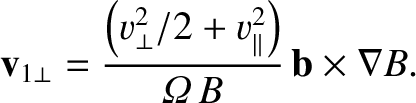(202)

It is easily verified that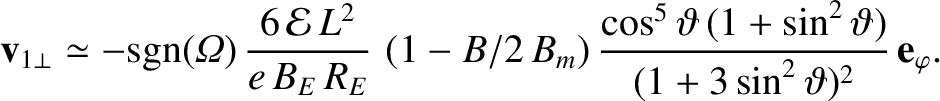(203)

Thus, the collisional energy conservation law can be written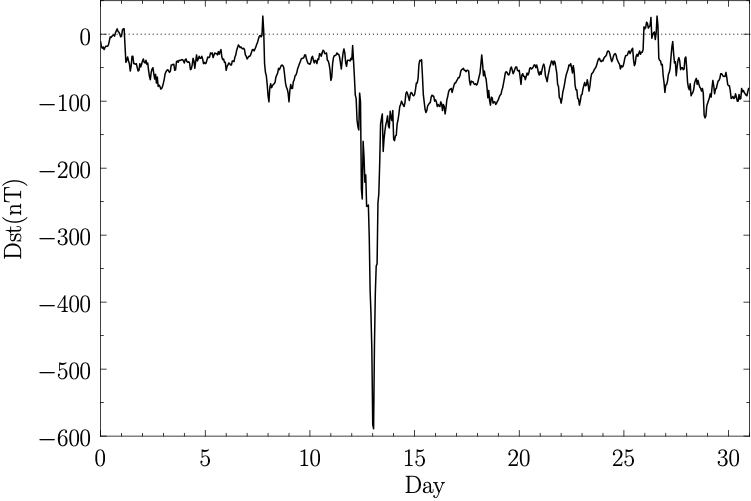(204)

or in non-detailed form(205)Next: Moments of the Kinetic Up: Plasma Fluid Theory Previous: Moments of the Distribution
Richard Fitzpatrick 2011-03-31